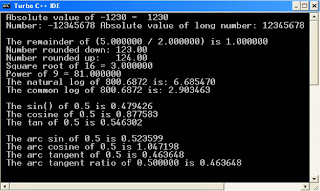This example in C Programming to demonstrates how to use some mathematics function like [acos(), asin(), atan(), atan2(), cos(), sin(), tan(), log(), log10(), pow(), sqrt(), ceil(), floor(), fmod(), abs(), labs()]. - WAY2IT

## Latest Post :Command to be Used :-
1. acos()
2. asin()
3. atan()
4. atan2()
5. cos()
6. sin()
7. tan()
8. log()
9. log10()
10. pow()
11. sqrt()
12. ceil()
13. floor()
14. fmod()
15. abs()
16. labs()

Code :-

#include<stdio.h>
#include<conio.h>
#include<math.h>
void main()
{
int non_abs=-1230;
long result;
long x = -12345678L;
double d1 = 5.0, d2 = 2.0;
double number = 123.54;
double down, up;
double at1, at2;
clrscr();
printf(" Absolute value of %d = %d",non_abs, abs(non_abs));
result= labs(x);
printf("\n Number: %ld Absolute value of long number: %ld\n", x, result);
printf("\n The remainder of (%lf / %lf) is %lf", d1, d2, fmod(d1,d2));
down = floor(number);
up = ceil(number);
printf("\n Number rounded down: %5.2lf", down);
printf("\n Number rounded up: %5.2lf", up);
printf("\n Square root of 16 = %lf", sqrt(9));
printf("\n Power of 9 = %lf", pow(9,2));
printf("\n The natural log of 800.6872 is: %lf", log(800.6872));
printf("\n The common log of 800.6872 is: %lf\n", log10(800.6872));
printf("\n The sin() of 0.5 is %lf", sin(0.5));
printf("\n The cosine of 0.5 is %lf", cos(0.5));
printf("\n The tan of 0.5 is %lf\n", tan(0.5));
printf("\n The arc sin of 0.5 is %lf", asin(0.5));
printf("\n The arc cosine of 0.5 is %lf", acos(0.5));
printf("\n The arc tangent of 0.5 is %lf", atan(0.5));
at1 = 90.0; at2 = 45.0;
printf("\n The arc tangent ratio of %lf is %lf", (at2 / at1), atan2(at2, at1));
getch();
}

Output :-